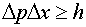The Heisenberg uncertainty principle In Schroedinger's equation the momentum was replaced by a derivative. This means that if a wave function is confined to a small volume, it must rise from zero quickly. Small volumes necessarily mean high momenta. This is analagous to the DeBroglie wavelength being inversely related to the momentum. Werner Heisenberg showed that one consequence of the Schroedinger equation is the Heisenberg uncertainty principlewhere Dx and Dp are the uncertainty in the momentum and position. The more confined a particle is to a given position, the more uncertain is the momentum. Thus a particle in its lowest energy state in a box of finite size has a range of momentum and therefore a finite kinetic energy. Similarly, an electron in a attractive Coulomb potential (e.g. a Hydrogen atom) must have more kinetic energy for smaller orbits. If it were not for this aspect of Schroedinger's equation, atoms could collapse to a point.